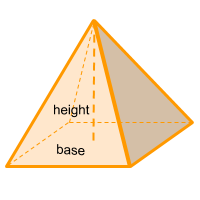Home » Polyhedra » Pyramids » Rectangular Pyramid

# Volume of a Rectangular PyramidVolume =
Area base × Height/3

## Volume calculator for a rectangular pyramid

Enter the height of the pyramid (Height) and the area of the base of the pyramid (area base) to get the volume automatically.

If you don't know the area of the base, enter the height of the pyramid (Height), the height of the rectangle that forms the base of the pyramid (height r) and the base of the rectangle that forms the base of the pyramid (base r) to get the volume automatically.

## Description, how many faces, edges and vertices are there in a rectangular pyramid

The rectangular pyramid, is a pyramid with a rectangle-shaped base. Just like the square pyramid, it has 5 faces, 4 faces are triangles that form the sides of the pyramid and one face that forms the base, which in this case is a rectangle; it has 5 vertices and 8 edges.

In case the base is a square (all sides are the same length), then we are in the presence of a square pyramid and not a rectangular one. Although the formula is the same.

## Formula for the volume of a rectangular pyramid

To calculate the volume of a rectangular pyramid, we must first know the area of its base. To get the value of the base we need to multiply the base of the rectangle by the height of the rectangle.
Once we know the area of the base (area of the rectangle), we multiply it by the height of the pyramid and finally we divide the result by 3. You can also use the online calculator to calculate the volume of the rectangular pyramid automatically.

Volume =
Area base × Height/3

Area base = Area rectangle = baser × heightr

Share